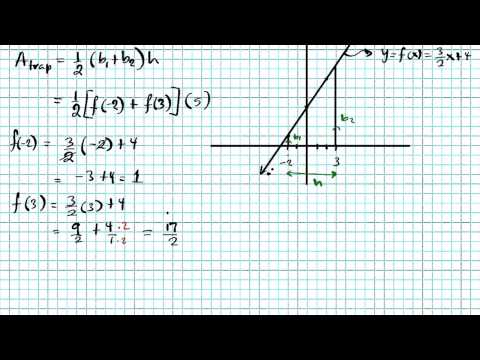# maths gotserved► Play all

# Integration techniques

In this playlist we will be reviewing the definite integral from multiple aspects.
We will start by looking at rectangular approximations, LRAM, MRAM, and RRAM. Then we will look at reimann sums expressed as limits. Convert the expression into integrals and evaluate using geometry. We will then look at rules for definite integrals.
In this playlist we will be reviewing the definite integral from multiple aspects.
We will start by looking at rectangular approximations, LRAM, MRAM, and RRAM. Then we will look at reimann sums expressed as limits. Convert the expression into in...